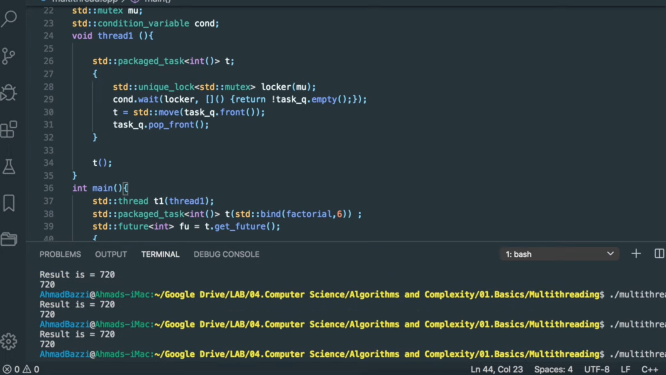GFG App
Open AppBrowser
Continue

The  std::packaged_task class wraps any Callable objects (function, lambda expression, bind expression, or another function object) so that they can be invoked asynchronously. A packaged_task won’t start on its own, you have to invoke it, As its return value is stored in a shared state that can be called/accessed by  std::future objects.

The main advantage of a packaged task is that it can link a callable object to a future and that is very important in a flooding environment. For example, if we have an existing function that fetches the data from Database (DB) and returns it. Now there is a need to execute this function in a separate thread. This can be done using:

Otherwise, we’ll have to use:

std::promise<>

and have to change code but with the help of  std::packaged_task<> its simple and we don’t need to do that.

Member Functions

Some of the member functions in packaged_task are:

• Operator=- it moves packaged tasks and it’s a public member function.
• Swap- It just swaps to the packaged task or you can say exchange two packaged tasks with each other.
• get_future- It returns a std::future associated with the promised result.
• reset- This public member function just resets the task.
• (constructor)- As the name suggests this public member function constructs the packaged task.
• (destructor)- Similarly, (destructor) destructs the task object.

Non-Member Functions

One of the non-member functions is:

• swap(packaged_task)- It specializes the std::swap algorithm.

Below is the C++ program to implement the above functions-

## C++

 `// C++ program to implement ` `// the functions ` `#include ` ` `  `using` `namespace` `std; ` ` `  `// Factorial function ` `int` `factorial(``int` `N) ` `{ ` `    ``int` `res = 1; ` `    ``for` `(``int` `i = N; i > 1; i--)  ` `    ``{ ` `        ``res *= i; ` `    ``} ` `   `  `    ``cout << ``"Result is = "` `<<  ` `             ``res << ``"/n"``; ` `    ``return` `res; ` `} ` ` `  `// packaged task ` `std::deque > task_q;     ` `std::mutex mu; ` `std::condition_variable cond; ` ` `  `void` `thread1()  ` `{ ` `  ``// packaged task ` `  ``std::packaged_task<``int``()> t;                        ` `  ``{ ` `    ``std::unique_lock locker(mu); ` `    ``cond.wait(locker, []()  ` `              ``{  ` `                ``return` `!task_q.empty(); ` `              ``});  ` `    ``t = std::move(task_q.front()); ` `    ``task_q.pop_front(); ` `  ``}   ` `  ``t(); ` `} ` ` `  `// Driver Code ` `int` `main() ` `{ ` `      ``std::``thread` `t1(thread1); ` `     `  `    ``// Create a packaged_task<> that  ` `    ``// encapsulated the callback i.e. a function ` `    ``std::packaged_task<``int``()> t(bind(factorial,6));     ` `   `  `    ``// Fetch the associated future<>  ` `    ``// from packaged_task<> ` `      ``std::future<``int``> fu = t.get_future();               ` `  ``{ ` `    ``std::lock_guard locker(mu); ` `    ``task_q.push_back(std::move(t)); ` `  ``} ` `   `  `      ``cond.notify_one(); ` `   `  `    ``// Fetch the result of packaged_task<> ` `    ``cout<

Output:My Personal Notes arrow_drop_up